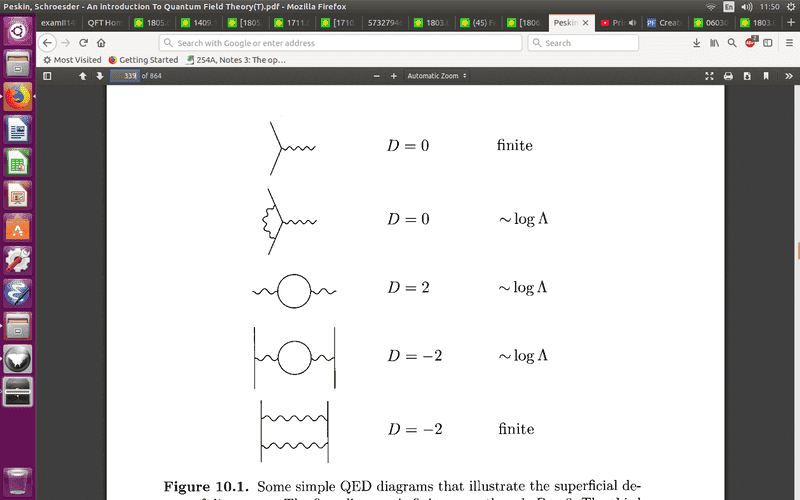# The superficial degree of divergence in Peskin and Schroeder

• MathematicalPhysicist

Gold Member

## Homework Statement

I have in the picture attached a screenshot from Peskin's textbook.

I don't understand how did they get that for the two last diagrams that ##D=-2##.
The question is from pages 316-317 of Peskin's textbook.## Homework Equations

$$D=4-N_{\gamma}-3/2N_e$$
where ##N_e##=number of external electron lines;
##N_\gamma##=number of external photon lines.

## The Attempt at a Solution

It seems that for the two last diagrams, we have: ##N_{\gamma}=N_e=2##, which means that ##D=4-2-2*3/2=2-3=-1##, why is it that ##D=-2##?

Last edited:

## Homework Statement

I have in the picture attached a screenshot from Peskin's textbook.

I don't understand how did they get that for the two last diagrams that ##D=-2##.
The question is from pages 316-317 of Peskin's textbook.View attachment 226716

## Homework Equations

$$D=4-N_{\gamma}-3/2N_e$$
where ##N_e##=number of external electron lines;
##N_\gamma##=number of external photon lines.

## The Attempt at a Solution

It seems that for the two last diagrams, we have: ##N_{\gamma}=N_e=2##, which means that ##D=4-2-2*3/2=2-3=-1##, why is it that ##D=-2##?
When they say that ##N_e## is the number of external fermion lines, it means the number of external incoming fermion lines plus th number of outgoing fermion lines. In the last two diagrams, ##N_e=4##.

When they say that ##N_e## is the number of external fermion lines, it means the number of external incoming fermion lines plus th number of outgoing fermion lines. In the last two diagrams, ##N_e=4##.
So ##N_\gamma=0##, why is that? I see two external photon lines.

Is it because the photon lines are connected to the electron lines?

Is it because the photon lines are connected to the electron lines?

Yes. Note that in the first diagrams you had to use ##N_e=2##